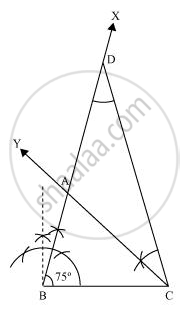# Construct a triangle ABC in which BC = 7 cm, ∠B = 75° and AB + AC = 13 cm. - Mathematics

Construct a triangle ABC in which BC = 7 cm, ∠B = 75° and AB + AC = 13 cm.

#### Solution

The below given steps will be followed to construct the required triangle.

Step I:- Draw a line segment BC of 7 cm. At point B, draw an angle of 75°, say ∠XBC.

Step II:- Cut a line segment BD = 13 cm (that is equal to AB + AC) from the ray BX.

Step III:- Join DC and make an angle DCY equal to ∠BDC.

Step IV:- Let CY intersect BX at A. ΔABC is the required triangle.Concept: Some Constructions of Triangles
Is there an error in this question or solution?
Chapter 11: Constructions - Exercise 11.2 [Page 195]

#### APPEARS IN

NCERT Class 9 Maths
Chapter 11 Constructions
Exercise 11.2 | Q 1 | Page 195

Share